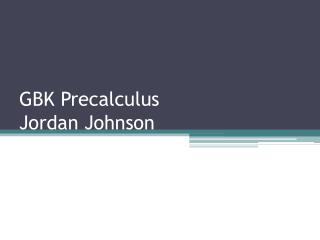DownloadDownload PresentationGBK Precalculus Jordan Johnson

GBK Precalculus Jordan Johnson

Download PresentationGBK Precalculus Jordan Johnson

- - - - - - - - - - - - - - - - - - - - - - - - - - - E N D - - - - - - - - - - - - - - - - - - - - - - - - - - -
Presentation Transcript

1. GBK PrecalculusJordan Johnson

2. Today’s agenda • Greetings • Conclude NaQ; Review • Lesson: Circular Functions (Sec. 3-5) • Homework • Clean-up

3. Not a Quiz • Put everything away except pen/pencil & calculator. • Part I is on the paper I’m handing out. • Do Part II on the back: • Copy and complete this table:

4. Problem 1: Period = 360°/4 = 90° ¼ cycle = 90°/4 = 22.5°

5. Solutions • y = ½ + 3.5 cos 0.9( – 200°) • y = -60 + 40 sin 7.5( – 36°) • Point of inflection:Increasing but concave-down: • y = 55 + 45 cos 12( + 3°) • y = 55 + 45 sin 12( – 19.5°)

6. Circular Functions • Main ideas of Sec. 3-5: • sin, cos, tan, etc. are functionsfrom angles (typically in degrees)to lengths (based on the unit circle). • “Circular functions” are the trig functions, redefined without using angles; they are functions from lengths to lengths.

7. Trigonometric Functions • Original definition of sin, cos, and tan: • Given an angle θ in standard position, whose terminal side intersects a circle of radius r at the point (u,v), we define: • sin θ = v/r • cos θ = u/r • tan θ = v/u

8. Circular Functions • Given an arc of length x on the unit circle, from (1,0) counterclockwise to (u,v), we define the circular functions as: • sin x = v • cos x = u • tan x = sin x/cos x • cot x = cos x/sin x • sec x = 1/cos x • csc x = 1/sin x

9. Circular Functions • cot x = cos x/sin x • sec x = 1/cos x • csc x = 1/sin x • sin x = v • cos x = u • tan x = sin x/cos x • These are equivalent to the trig functions. • If the argument is x (or another Roman letter),assume it’s in radians. • If the argument is  (or another Greek letter),assume it’s in degrees.

10. Homework • Skim §3-5 (pp. 117-121) and: • Answer Q1-Q10. • Do Exercises 1-5, 7, 10, 14, 19, 23, 27, 31, 39, 44. • Due Monday, 11/8. • Next assignment will be from §3-6 (pp. 124-28): • Read it, and answer Reading Analysis questions. • Answer Q1-Q10. • Do Exercises 1-13 odd.

11. Clean-up / Reminders • Pick up all trash / items. • Push in chairs (at front and side tables). • See you tomorrow!